﻿ Exergoeconomic Analysis of a Boiler for a Coal Fired Thermal Power Plant

### Exergoeconomic Analysis of a Boiler for a Coal Fired Thermal Power Plant

Mukesh Gupta, Raj KumarOPEN ACCESSPEER-REVIEWED

## Exergoeconomic Analysis of a Boiler for a Coal Fired Thermal Power Plant

Mukesh Gupta1,, Raj Kumar1

1Department of Mechanical Engineering, YMCA University of Science & Technology, Faridabad, India

### Abstract

The purpose of this study is to analyze comparatively the performance of a boiler used in a coal fired thermal power plant, from exergoeconomic viewpoint. Firstly, thermodynamic models of the plants are developed based on second law of thermodynamics. Secondly design point performance analyses based on exergetic and exergoeconomic performance criteria such as cost of exergetic destruction, relative cost difference and exergoeconomic factor are performed for the boiler used in a 55 MW power plant using an example in order to make comprehensive evaluations. As a result, the outcomes of this study can provide a basis used for boiler performance analysis and improvement.

### At a glance: Figures

12
Prev Next

• Gupta, Mukesh, and Raj Kumar. "Exergoeconomic Analysis of a Boiler for a Coal Fired Thermal Power Plant." American Journal of Mechanical Engineering 2.5 (2014): 143-146.
• Gupta, M. , & Kumar, R. (2014). Exergoeconomic Analysis of a Boiler for a Coal Fired Thermal Power Plant. American Journal of Mechanical Engineering, 2(5), 143-146.
• Gupta, Mukesh, and Raj Kumar. "Exergoeconomic Analysis of a Boiler for a Coal Fired Thermal Power Plant." American Journal of Mechanical Engineering 2, no. 5 (2014): 143-146.

 Import into BibTeX Import into EndNote Import into RefMan Import into RefWorks

### 1. Introduction

A boiler is an enclosed vessel that provides a means for combustion heat to be transferred into water until it becomes heated water or steam. The hot water or steam under pressure is then usable for transferring the heat to a process. The heating surface is any part of the boiler metal that has hot gases of combustion on one side and water on the other. Any part of the boiler metal that actually contributes to making steam is heating surface. The amount of heating surface of a boiler is expressed in square meters.

The water supplied to the boiler that is converted into steam is called feed water. The two sources of feed water are: (1) Condensate or condensed steam returned from the processes and (2) Makeup water (treated raw water) which must come from outside the boiler room and plant processes. For higher boiler efficiencies, the feed water is preheated by economizer, using the waste heat in the flue gas.

The combination of exergetic and economic analysis called exergoeconomic analysis has become a powerful tool for assessing the performance of energy conservation systems. In this series many researchers have taken keen interest to prove the importance of this technique. Exergoeconomics has been effectively used as a design tool for the realization of a gas turbine power plant principle . Optimization of a single and double effect vapour absorption refrigeration system has been done with good results [2, 3, 4]. Optimization of a cogeneration system using exergoeconomic technique has provided excellent results . Exergoeconomic analysis has been applied to different kinds of thermal systems with varying degree of success [6, 7, 8]. Use of exergetic cost theory and symbolic exergoeconomics provide an effective tool to analyse and optimize thermal systems [9, 10]. Variables such as exergetic efficiency, the rates of exergy destruction, exergy destruction ratio, and the cost rates associated with exergy destruction, capital investment, operation and maintenance cost, relative cost difference of unit costs and exergoeconomic factor provide a holistic view of the operation of the plant . Several thermodynamic relations between the energy and exergy losses and capital cost for thermal systems in a modern coal fired electrical generation station have been developed and used extensively in research [12-16].

### 2. Methodology

2.1. Boiler System

The boiler system comprises of feed water system, steam system and fuel system. The feed water system provides water to the boiler and regulates it automatically to meet the steam demand. Various valves provide access for maintenance and repair. The steam system collects and controls the steam produced in the boiler. Steam is directed through a piping system to the point of use. Throughout the system, steam pressure is regulated using valves and checked with steam pressure gauges. The fuel system includes all equipment used to provide fuel to generate the necessary heat. The equipment required in the fuel system depends on the type of fuel used in the system. A typical boiler room schematic is shown in Figure 1.

2.2. Exergy Analysis

The major streams entering and leaving the boiler are shown in Figure 1. A block diagram for the same is developed as shown in Figure 2.

The general energy and exergy balance equations for the boiler may be formulated using the first and second law of thermodynamics. The specific thermo- mechanical exergy (neglecting kinetic and potential exergy) is evaluated from Eq. (1):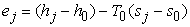(1)

The total rate of exergy with any stream can be estimated as given in Eq. (2)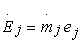(2)

The total rate of energy with any stream is given by Eq. (3).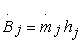(3)

The exergy flow rate leaving the flue gas is given by Eq. (4).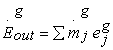(4)

The exergy balance equation for boiler is given as in Eq. (5).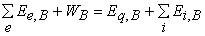(5)

The exergy destruction ratio yD,B for the boiler is represented as in Eq. (6).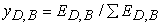(6)

The exergy loss ratio for the boiler is given by Eq. (7).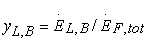(7)

The exergetic efficiency of a boiler is given by Eq. (8).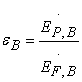(8)
2.3. Exergoeconomic Analysis

The generalized cost balance equation for a boiler is given as in Eq. (9).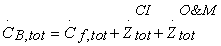(9)

The cost balance equation for a boiler system represented by Figure 2 is given by Eq. (10).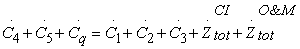(10)

Average costs per exergy unit of fuel and product for a boiler are given as per Eq. (11) and Eq. (12).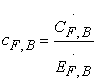(11)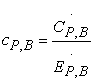(12)
2.4. Thermoeconomic Variables
2.4.1. Cost of Exergy Destruction

For determining the cost of exergy destruction two approaches are generally used. In the first approach the product from the boiler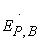is assumed to be fixed and the cost of exergy destruction is given by Eq. (13).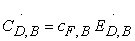(13)

In this approach the fuel rate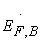must account for the fixed product rate and the rate of exergy destruction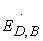. Alternatively assuming that the fuelis fixed the cost of exergy destruction is formulated as in Eq. (14).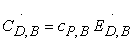(14)

2.4.2. Relative Cost Difference

The relative cost difference for a boiler reveals the real cost associated with it and is given by Eq. (15).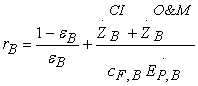(15)

2.4.3. Exergoeconomic Factor

The exergoeconomic factor gives the relative significance of the two categories of costs associated with a boiler namely, non-exergy-related costs (capital investment & operation & maintenance cost) and exergy related costs (cost of exergy destruction and exergy losses). It is given by Eq. (16).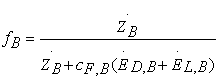(16)

### 3. Illustrative Example

To illustrate the benefits of the exergoeconomic technique a boiler for a 55 MW coal fired thermal power plant is considered for which the following data is available

•  Coal received is of 16765 KJ/kg calorific value with ash content of 38% and 26% volatile matter.

•  For normal running of plant at 55MW, boiler generates the 72.22 kg/s of superheated steam at 93.17 bars.

Calculations are done with following assumptions

1) Specific exergy of fuel has been calculated as in .

2) Gross calorific value has been used in calculations.

3) Intensive properties of the environment are not changing due to any process.

#### Table 1. Pressure, temperature, mass flow rate and exergy flow rate for boiler streams of 55 MW Power Plant

The analysis of actual boiler system and its components is highly complex and tedious in nature due to huge amount of data associated with large number of system components, different operating conditions and nonlinear behaviour of steam properties.

The various costs associated with the functioning of a boiler are given in Table 2.

#### Table 2. Initial investment costs, annualized costs and corresponding monetary flow rates of boiler in a 55MW power plant

The values for exergy, cost rates associated with flows and the unit costs of flows for the boiler are given in Table 3.

### 4. Results & Discussion

The boiler has been analyzed from exergetic and thermoeconomic considerations. Variation of various thermoeconomic factors have been calculated for variations of temperature and pressure.

Table 1 illustrates the values for pressure, temperature, mass flow rate and exergy flow rate for the various boiler streams. Table 3 gives the values for exergy and cost rates associated with flows and unit costs of flows for the boiler. Table 4 illustrates the values for PEC and the different thermoeconomic variables for the boiler.

Figure 3 indicates the effect of pressure variation on the values of the relative cost difference (rB) for the boiler. It shows that with the drop in pressure there is a drop in the values for the relative cost difference as well and this drop becomes more and more prominent as the pressure values reduce significantly.

Figure 4 indicates the effect of variation in pressure on the exergoeconomic factor (fB). The variation in the values of the exergoeconomic factor is significant for lower values of pressure.

Figure 5 indicates the effect of variation in temperature on the relative cost difference (rB). The present study illustrates that there exists an inverse relationship between the values of pressure and the corresponding relative cost difference.

Figure 6 indicates the effect of variation in temperature on the exergoeconomic factor (fB). The present study illustrates that there exists an inverse relationship between the values of pressure and the corresponding exergoeconomic factor.

Low values of the relative cost difference and exergoeconomic factor at lower temperatures and pressures indicate that cost savings can be achieved by reducing the exergy destruction rate even though it may require higher capital investment.

### 5. Conclusions

The values for various thermoeconomic variables are calculated and their values are as follows:

Unit Cost of Fuel Exergy cF (Rs/MJ) = 0.1061

Unit Cost of Product Exergy cP (Rs/MJ) = 0.2543

Cost flow Rate of Stream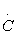(Rs/hr) = 99135.72

Cost of exergy destruction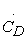(Rs/hr) = 505409.4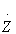(Rs/hr) = 292.36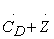(Rs/hr) = 292.36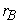= 1.6570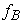= 0.0012

This paper proposes a novel approach to analyze the boiler system used in coal based thermal power plants using the second law of thermodynamics approach. The present analysis provides the following conclusions:

(1) The exergy analysis is more realistic than the conventional first law based energy approach.

(2) The exergy analysis provides a better insight into the working of the boiler and helps to assign direct monetary values to the different thermal factors.

(3) The present study provides a comprehensive analysis of variation of the different thermoeconomic factors with respect to temperature and pressure and hence it helps to determine the optimum values of temperature and pressure which can be used to obtain maximum efficiency from the boiler while keeping the costs down to minimum.

It can be concluded through the above discussion that exergy analysis is a much better way to analyse the working of a boiler used in a coal fired thermal power plant.

### References

  Attala, L., Facchini, B., Ferrara, G. Thermoeconomic optimization method design tool in gas-steam combined plant realization, Energy Conversion and Management 42 (2001), 18, pp. 2163-2172.In article  Mishra R.D., Sahoo P.K. and Gupta A., Application of exergetic cost theory to LiBr/H2O vapour absorption system, Energy, 2002, 1009-1025.In article CrossRef  Mishra R.D., Sahoo P.K. and Gupta A., Thermoeconomic optimization of single effect water/ LiBr vapour absorption refrigeration system, International Journal of Refrigeration, 2003, 26, 158-169.In article CrossRef  Mishra R.D., Sahoo P.K. and Gupta A., Thermoeconomic optimization of double effect water/ LiBr vapour absorption refrigeration system, International Journal of Refrigeration, 2005, 28, 331-343.In article CrossRef  Sahoo P.K., Exergoeconomic analysis and optimization of a cogeneration system using evolutionary programming, Applied Thermal Engineering, 2008, 28, 1580-1588.In article CrossRef  Lozano M.A. and Valero A., Theory of Exergetic cost, Energy, 1993, 18, 939-60.In article CrossRef  Bejan A., Tsatsaronis G. and Moran M., Thermal Design and Optimization, New York: Wiley, 1996.In article  Erlach B., Serra L. and Valero A., Structural theory as standard for thermoeconomics, Energy Conversion and Management, 1999, 40, 1627-1649.In article CrossRef  Valero A. and Torres C., On causality in organized energy systems: II. Symbolic exergoeconomics, University of Zaragoza, Spain.In article  Valero A., Lerch F., Serra L. and Royo J., Structural theory and thermoeconomic diagnosis: Part II, Application to actual power plant, Energy Conversion and Management, 2002, 43, 1519-1535.In article CrossRef  Moran M.J. and Sciubba E., Exergy analysis: Principles and practice, Journal of Engineering for Gas Turbines and Power, 1994,116, 285-290.In article CrossRef  Rosen M., Clarifying thermodynamic efficiencies and losses via exergy, Exergy International Journal, 2002, 2, 3-5.In article CrossRef  Rosen M., Editorial-Exergy in industry: Accepted or not?, Exergy, An International Journal. 2001, 2, 67.In article  Rosen M., Energy and Exergy based comparison of coal fired and nuclear steam power plants, Exergy International Journal, 2001, 3, 180-192.In article CrossRef  Rosen M., Energy crisis or exergy crisis? Exergy, An International Journal, 2002, 2, 125-127.In article  Rosen M. and Dincer I., Thermoeconomic, Analysis of power plants: an application to a coal fired electrical generating station, Energy Conversion and Management, 2003, 44, 2743-2761.In article CrossRef